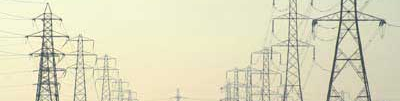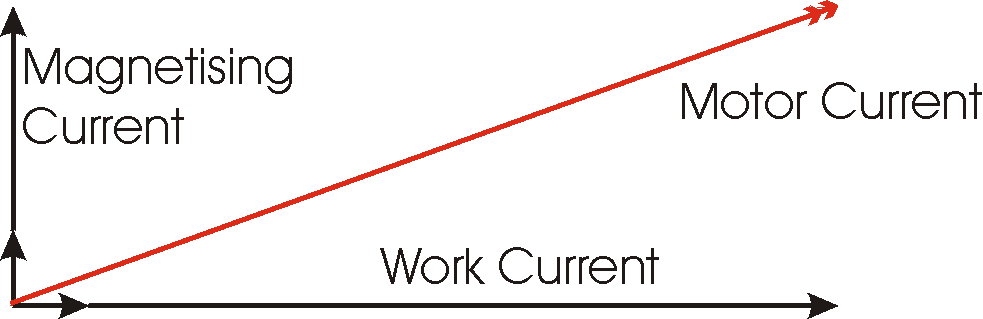# Displacement Power Factor

## What is Displacement Power Factor?

Displacement Power Factor is caused by a reactive component in the load. If there is an inductive compinent in the load, then there will be an inductive current flowing in addition to the resistive current. The inductive curent follows the voltage waveform by 90 degrees. Likewise a capacitive component causes a capacitive current that leads the voltage waveform by 90 degrees. The vector sum of the reactive (capacitive and/or inductive) current(s) and the resistive current results in a single current with a phase angle before (leading) or after(lagging) the voltage waveform. The displacment power factor value is the cosine of the angle between the voltage waveform and the resultant current waveform.
Displacement power factor is typically decreased by inductive loads such as induction motors, transformers and lighting ballasts.

## Power Factor of an Induction Motor

Reference to the equivilent circuitof an induction motor, shows that the induction motor draws current from the supply that is made up of resistive components and inductive components. The resistive components are: